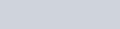Solve this
Question:

The $\mathrm{K}_{\mathrm{sp}}$ for $\mathrm{Cr}(\mathrm{OH})_{3}$ is $1.6 \times 10^{-30}$. The molar solubility of this compound in water is :-

1. $\sqrt{1.6 \times 10^{-30}}$

2. $\sqrt{1.6 \times 10^{-30}}$

3. $\sqrt{1.6 \times 10^{-30} / 27}$

4. $1.6 \times 10^{-30} / 27$

Correct Option: , 3

Solution: